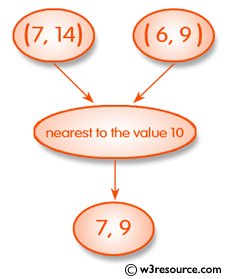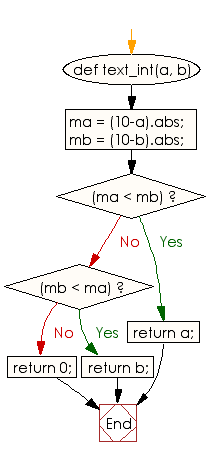﻿ Ruby Basic exercises: Check two integers and return whichever value is nearest to the value 10 - w3resource# Ruby Basic Exercises: Check two integers and return whichever value is nearest to the value 10

## Ruby Basic: Exercise-36 with Solution

Write a Ruby program to check two integers and return whichever value is nearest to the value 10, or return 0 if two integers are equal.Ruby Code:

``````def text_int(a, b)
ma = (10-a).abs;
mb = (10-b).abs;
if (ma < mb)
return a;
end
if (mb < ma)
return b;
end
return 0;
end
print text_int(7, 14),"\n"
print text_int(6, 9),"\n"
print text_int(5, 5)
``````

Output:

```7
9
0
```

Flowchart:Ruby Code Editor: